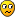## Stepper Hybrid - JP7 vs JP5

Moderators: TomKerekes, dynomotion

nice_kflop
Posts: 10
Joined: Tue Aug 31, 2021 11:47 pm

### Stepper Hybrid - JP7 vs JP5

I have successfully been able to move the closed loop stepper motor that uses a hybrid servo drive "HBS860H". When I have it hooked on JP7, i am able to move the motor by the kmotion "step response" screen and from the "kmotionCNC" jog buttons. But, when I use the JP5, I am only able to move the stepper from the "step response" screen but the "kmotionCNC" jog buttons are not moving it. Any suggestion?
................................
When using the JP7:
> PUL+ and DIR+ hooked to 5V pin 24(VDD5)
> PUL- hooked to pin 15
> DIR- hooked to pin 16
...............................
When using the JP5:
> PUL+ and DIR+ hooked to 5V pin 24(VDD5) from JP7
> PUL- hooked to pin 1/IO36
> DIR- hooked to pin 2/IO37
..............................

TomKerekes
Posts: 1935
Joined: Mon Dec 04, 2017 1:49 am

### Re: Stepper Hybrid - JP7 vs JP5

Hi,

Step/Dir Generators 4-7 are connected to KFLOP JP5

What KFLOP Axis are you using in the Step Response Screen when moving the Stepper?

What Jog Buttons in KMotionCNC are you expecting to move the Axis?

The DefineCoordSystem() function is used to assign KMotionCNC GCode Axes to KFLOP Axes. ie:

DefineCoordSystem(0,1,2,-1); // define X Y Z

How are you defining your axes?
Regards,

Tom Kerekes
Dynomotion, Inc.

nice_kflop
Posts: 10
Joined: Tue Aug 31, 2021 11:47 pm

### Re: Stepper Hybrid - JP7 vs JP5

Hi,
Thanks for your reply on DefineCoordSystem. I did change it please take a peak at the code below. I have 3 steppers attached to channels 0 x-axis, ch1 y-axis, and ch4 (assuming its a-axis)...channel 4 does move the stepper on the step response screen....in the axis window, i have 0, 1, and 4 enabled.
Reason why i jumpted to channel 4 is because i want to eventually use kflop for 5-axis, am trying to see if that fifth axis works. The jog buttons arrow buttons for A-axis on kmotionCNC screen dont move the stepper motor. Any suggestions please?
The encoder is connected to the hybrid servo drive "HBS860H".

Code: Select all

``````
#include "KMotionDef.h"

int main()
{
ch0->InputMode=ENCODER_MODE;
ch0->OutputMode=CL_STEP_DIR_MODE;
ch0->Vel=10000;
ch0->Accel=400000;
ch0->Jerk=4e+06;
ch0->P=0;
ch0->I=0.01;
ch0->D=0;
ch0->FFAccel=0;
ch0->FFVel=0;
ch0->MaxI=200;
ch0->MaxErr=1e+06;
ch0->MaxOutput=200;
ch0->InputChan0=0;
ch0->InputChan1=0;
ch0->OutputChan0=0;
ch0->OutputChan1=0;
ch0->MasterAxis=-1;
ch0->LimitSwitchOptions=0x100;
ch0->LimitSwitchNegBit=0;
ch0->LimitSwitchPosBit=0;
ch0->SoftLimitPos=1e+09;
ch0->SoftLimitNeg=-1e+09;
ch0->InputGain0=1;
ch0->InputGain1=1;
ch0->InputOffset0=0;
ch0->InputOffset1=0;
ch0->OutputGain=1;
ch0->OutputOffset=0;
ch0->SlaveGain=1;
ch0->BacklashMode=BACKLASH_OFF;
ch0->BacklashAmount=0;
ch0->BacklashRate=0;
ch0->invDistPerCycle=1;
ch0->MaxFollowingError=1000000000;
ch0->StepperAmplitude=20;

ch0->iir.B0=1;
ch0->iir.B1=0;
ch0->iir.B2=0;
ch0->iir.A1=0;
ch0->iir.A2=0;

ch0->iir.B0=1;
ch0->iir.B1=0;
ch0->iir.B2=0;
ch0->iir.A1=0;
ch0->iir.A2=0;

ch0->iir.B0=0.000769;
ch0->iir.B1=0.001538;
ch0->iir.B2=0.000769;
ch0->iir.A1=1.92081;
ch0->iir.A2=-0.923885;
EnableAxisDest(0,0);

ch1->InputMode=ENCODER_MODE;
ch1->OutputMode=CL_STEP_DIR_MODE;
ch1->Vel=10000;
ch1->Accel=400000;
ch1->Jerk=4e+06;
ch1->P=0;
ch1->I=0.01;
ch1->D=0;
ch1->FFAccel=0;
ch1->FFVel=0;
ch1->MaxI=200;
ch1->MaxErr=1e+06;
ch1->MaxOutput=200;
ch1->InputChan0=1;
ch1->InputChan1=0;
ch1->OutputChan0=1;
ch1->OutputChan1=0;
ch1->MasterAxis=-1;
ch1->LimitSwitchOptions=0x100;
ch1->LimitSwitchNegBit=0;
ch1->LimitSwitchPosBit=0;
ch1->SoftLimitPos=1e+09;
ch1->SoftLimitNeg=-1e+09;
ch1->InputGain0=1;
ch1->InputGain1=1;
ch1->InputOffset0=0;
ch1->InputOffset1=0;
ch1->OutputGain=1;
ch1->OutputOffset=0;
ch1->SlaveGain=1;
ch1->BacklashMode=BACKLASH_OFF;
ch1->BacklashAmount=0;
ch1->BacklashRate=0;
ch1->invDistPerCycle=1;
ch1->MaxFollowingError=1000000000;
ch1->StepperAmplitude=20;

ch1->iir.B0=1;
ch1->iir.B1=0;
ch1->iir.B2=0;
ch1->iir.A1=0;
ch1->iir.A2=0;

ch1->iir.B0=1;
ch1->iir.B1=0;
ch1->iir.B2=0;
ch1->iir.A1=0;
ch1->iir.A2=0;

ch1->iir.B0=0.000769;
ch1->iir.B1=0.001538;
ch1->iir.B2=0.000769;
ch1->iir.A1=1.92081;
ch1->iir.A2=-0.923885;
EnableAxisDest(1,0);

ch4->InputMode=ENCODER_MODE;
ch4->OutputMode=CL_STEP_DIR_MODE;
ch4->Vel=10000;
ch4->Accel=400000;
ch4->Jerk=4e+06;
ch4->P=0;
ch4->I=0.01;
ch4->D=0;
ch4->FFAccel=0;
ch4->FFVel=0;
ch4->MaxI=200;
ch4->MaxErr=1e+06;
ch4->MaxOutput=200;
ch4->InputChan0=2;
ch4->InputChan1=0;
ch4->OutputChan0=2;
ch4->OutputChan1=0;
ch4->MasterAxis=-1;
ch4->LimitSwitchOptions=0x100;
ch4->LimitSwitchNegBit=0;
ch4->LimitSwitchPosBit=0;
ch4->SoftLimitPos=1e+09;
ch4->SoftLimitNeg=-1e+09;
ch4->InputGain0=1;
ch4->InputGain1=1;
ch4->InputOffset0=0;
ch4->InputOffset1=0;
ch4->OutputGain=1;
ch4->OutputOffset=0;
ch4->SlaveGain=1;
ch4->BacklashMode=BACKLASH_OFF;
ch4->BacklashAmount=0;
ch4->BacklashRate=0;
ch4->invDistPerCycle=1;
ch4->MaxFollowingError=1000000000;
ch4->StepperAmplitude=250;

ch4->iir.B0=1;
ch4->iir.B1=0;
ch4->iir.B2=0;
ch4->iir.A1=0;
ch4->iir.A2=0;

ch4->iir.B0=1;
ch4->iir.B1=0;
ch4->iir.B2=0;
ch4->iir.A1=0;
ch4->iir.A2=0;

ch4->iir.B0=0.000769;
ch4->iir.B1=0.001538;
ch4->iir.B2=0.000769;
ch4->iir.A1=1.92081;
ch4->iir.A2=-0.923885;
EnableAxisDest(4,0);

DefineCoordSystem(0,1,4,-1);

return 0;
}
``````

TomKerekes
Posts: 1935
Joined: Mon Dec 04, 2017 1:49 am

### Re: Stepper Hybrid - JP7 vs JP5

You defined KFLOP Axis #4 as the Z axis. If you want it as the A axis code:

Code: Select all

``DefineCoordSystem(0,1,-1,4);``
Note KFLOP Axis #4 is configured so use Step/Dir Generator #2 which is on KFLOP JP7 not JP5. I thought you were trying to test using KFLOP JP5?
Regards,

Tom Kerekes
Dynomotion, Inc.

nice_kflop
Posts: 10
Joined: Tue Aug 31, 2021 11:47 pm

### Re: Stepper Hybrid - JP7 vs JP5

Hi, yes i wanted to test JP5, just now i got it working on JP5 after your first post here about DefineCoordSystem, all i had to do is ground the other wires from pins 5 to 7 on jp5 and change the DefineCoordSystem. The jog works now. Thank you.

TomKerekes
Posts: 1935
Joined: Mon Dec 04, 2017 1:49 am

### Re: Stepper Hybrid - JP7 vs JP5

Its not clear what you did but there shouldn't be any reason to ground JP5 pins and may cause damage.
Regards,

Tom Kerekes
Dynomotion, Inc.

nice_kflop
Posts: 10
Joined: Tue Aug 31, 2021 11:47 pm

### Re: Stepper Hybrid - JP7 vs JP5AttachmentsTomKerekes
Posts: 1935
Joined: Mon Dec 04, 2017 1:49 am

### Re: Stepper Hybrid - JP7 vs JP5

No need to do that. Just ignore numbers not being used from inputs left floating. But it is ok if it bothers you as long as you don't set those outputs high.

Note please post wiring diagrams not photos.
Regards,

Tom Kerekes
Dynomotion, Inc.

nice_kflop
Posts: 10
Joined: Tue Aug 31, 2021 11:47 pm

### Re: Stepper Hybrid - JP7 vs JP5

I read the manual and tried so many configurations, my encoder has 1000 lines and i set the micro stepping drive to 4000 pulse/rev. Do i leave it as is in the image. What changes should I make.
- Also,channel 4 has same motor and driver as channel 1 but graph looks much different. Is that because of the amplitudeTomKerekes
Posts: 1935
Joined: Mon Dec 04, 2017 1:49 am

### Re: Stepper Hybrid - JP7 vs JP5

The encoders are not working. Channel 0 is doing nothing. Channel 4 is just counting +1, -1, +1, -1,... as if the A or B channel wasn't connected or working.
Regards,

Tom Kerekes
Dynomotion, Inc.